JEE  >  JEE Previous Year Questions (2021-22): Communication Systems

# JEE Previous Year Questions (2021-22): Communication Systems - Physics 35 Year Past year Papers JEE Main & Advanced

Q.1. A modulating signal 2sin⁡(6.28 × 106)t is added to the carrier signal 4sin⁡(12.56 × 109)t for amplitude modulation. The combined signal is passed through a non-linear square law device. The output is then passed through a band pass filter. The bandwidth of the output signal of band pass filter will be _________ MHz. [JEE Main 2022]

Ans. 2
W= 12.56 × 109
Wm  =6.25 × 106
After amplitude modulation
Bandwidth frequency
=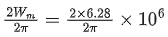= 2MHz

Q.2. The required height of a TV tower which can cover the population of 6.03 lakh is h. If the average population density is 100 per square km and the radius of earth is 6400 km, then the value of h will be _____________ m. [JEE Main 2022]

Ans. 150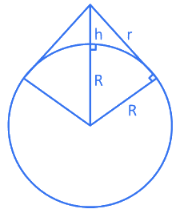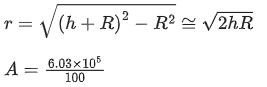πr= 6.03 × 103
π2Rh = 6.03 × 103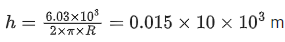= 150 m

Q.3. An antenna is placed in a dielectric medium of dielectric constant 6.25. If the maximum size of that antenna is 5.0 mm, it can radiate a signal of minimum frequency of __________ GHz.
(Given μr = 1 for dielectric medium) [JEE Main 2022]

Ans. 6
We know that v = fλ
Putting the values,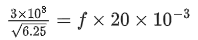⇒ f = 6 × 109 Hz

Q.4. The height of a transmitting antenna at the top of a tower is 25 m and that of receiving antenna is, 49 m. The maximum distance between them, for satisfactory communication in LOS (Line-Of-Sight) is K√5 × 102 m. The value of K is ___________. [JEE Main 2022]
(Assume radius of Earth is 64 × 10+5 m) [Calculate upto nearest integer value]

Ans. 192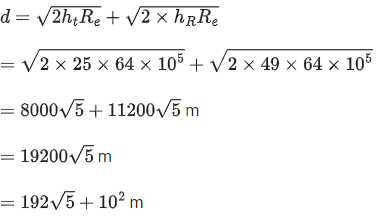Q.5. Find the modulation index of an AM wave having 8 V variation where maximum amplitude of the AM wave is 9 V. [JEE Main 2022]
(a) 0.8
(b) 0.5
(c) 0.2
(d) 0.1

Ans. a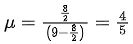= 0.8

Q.6. A FM Broadcast transmitter, using modulating signal of frequency 20 kHz has a deviation ratio of 10. The Bandwidth required for transmission is : [JEE Main 2022]
(a) 220 kHz
(b) 180 kHz
(c) 360 kHz

(d) 440 khz

Ans. d
Bandwidth of FM wave = 2(Δf + fm)
Δf / fm = 10 (Given)
Δf = fm(10) = 20 × 10 = 200 kHz
BW = 2(200 + 20) kHz
= 440 kHz

Q.7. In the case of amplitude modulation to avoid distortion the modulation index (μ) should be: [JEE Main 2022]
(a) μ ≤ 1
(b) μ ≥ 1
(c) μ = 2
(d) μ = 0

Ans. a
For effective modulation,
μ  ≤  1

Q.8. A square wave of the modulating signal is shown in the figure. The carrier wave is given by C(t)=5sin⁡(8πt) Volt. The modulation index is : [JEE Main 2022]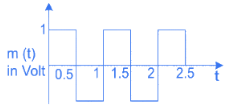(a) 0.2
(b) 0.1
(c) 0.3
(d) 0.4

Ans. a
μ = Am / Ac
= 1/5 = 0.2

Q.9. At a particular station, the TV transmission tower has a height of 100 m. To triple its coverage range, height of the tower should be increased to [JEE Main 2022]
(a) 200 m
(b) 300 m
(c) 600 m
(d) 900 m

Ans. d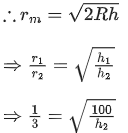⇒ h2 = 900 m

Q.10. The maximum and minimum voltage of an amplitude modulated signal are 60 V and 20 V respectively. The percentage modulation index will be : [JEE Main 2022]
(a) 0.5%
(b) 50%

(c) 2%
(d) 30%

Ans. b
Percentage modulation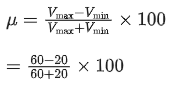= 50%

Q.11. A radio can tune to any station in 6MHz to 10MHz band. The value of corresponding wavelength bandwidth will be : [JEE Main 2022]
(a) 4 m
(b) 20 m

(c) 30 m
(d) 50 m

Ans. b
v1 = 6 × 106 Hz
⇒ λ1= 3 × 10/ 6 × 106 = 50 m
v2=10 × 106 Hz
⇒ λ2 = 3 × 108/ 10 × 10= 30 m
⇒ Wavelength band with
=|λ1−λ2|=20 m

Q.12. In AM modulation, a signal is modulated on a carrier wave such that maximum and minimum amplitudes are found to be 6 V and 2 V respectively. The modulation index is:
[JEE Main 2022]
(a) 100%
(b)80%
(c) 60%
(d) 50%

Ans. d
Amax = 6 V
Amin = 2 V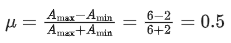μ = 50%

Q.13. A speech signal given by 11 sin(2200 πt) V is used for amplitude modulation with a carrier signal given by 44 sin(6600 πt) V. The minimum amplitude of modulated wave will be:
[JEE Main 2022]
(a) 33 V
(b) 55 V
(c) 8.25 V
(d) 13.75 V

Ans. a
Amin = AC − Am
= (44 - 11) volt
= 33 volt

Q.14. A baseband signal of 3.5 MHz frequency is modulated with a carrier signal of 3.5 GHz frequency using amplitude modulation method. What should be the minimum size of antenna required to transmit the modulated signal? [JEE Main 2022]
(a) 42.8 m
(b) 42.8 mm
(c) 21.4 mm
(d) 21.4 m

Ans. c
vc = 3.5 × 109 Hz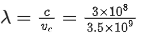∴ Size of antenna =λ / 4
=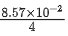= 21.4 mm

Q.15. A signal of 100 THz frequency can be transmitted with maximum efficiency by :
[JEE Main 2022]
(a) Coaxial cable
(b) Optical fibre
(c) Twisted pair of copper wires

(d) Water

Ans. b
Optical fibres supports frequency of electromagnetic waves in the range 1014 Hz to 1015 Hz.

Q.16. Match List I with List II [JEE Main 2022]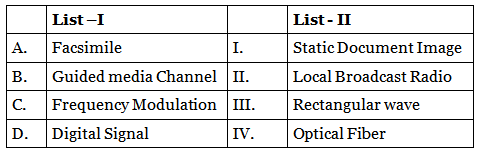Choose the correct answer from the following options:
(a) A-IV, B-III, C-II, D-I
(b) A-I, B-IV, C-II, D-III
(c) A-IV, B-II, C-III, D-I
(d) A-I, B-II, C-III, D-IV

Ans. b
The correct match is :
Facsimile    -    Static Document Imag
Guided Media Channel    -    Optical Fibe
Digital single    -    Rectangular Wave

Q.17. A sinusoidal wave y(t) = 40sin(10 × 106 πt) is amplitude modulated by another sinusoidal wave x(t) = 20sin (1000 πt). The amplitude of minimum frequency component of modulated signal is : [JEE Main 2022]
(a) 0.5
(b) 0.25
(c) 20
(d) 10

Ans. d
Modulate signal s(t) ≡ [1 + 20sin⁡(1000πt)]sin⁡(107πt)
≡ sin⁡(107πt) + 10cos⁡(107πt − 103πt) + 10cos⁡(107πt + 103πt)
⇒ Required amplitude = 10

Q.18. Choose the correct statement for amplitude modulation : [JEE Main 2022]
(a) Amplitude of modulating signal is varied in accordance with the information signal.
(b) Amplitude of modulated signal is varied in accordance with the information signal.
(c) Amplitude of carrier signal is varied in accordance with the information signal.
(d) Amplitude of modulated signal is varied in accordance with the modulating signal.

Ans. c
In amplitude modulation, amplitude of carrier signal is varied according to the message signal.

Q.19. We do not transmit low frequency signal to long distances because-
(a) The size of the antenna should be comparable to signal wavelength which is unreal solution for a signal of longer wavelength.
(b) Effective power radiated by a long wavelength baseband signal would be high.
(c) We want to avoid mixing up signals transmitted by different transmitter simultaneously.
(d) Low frequency signal can be sent to long distances by superimposing with a high frequency wave as well.
Therefore, the most suitable option will be : [JEE Main 2022]
(a) All statements are true
(b) (a), (b) and (c) are true only
(c) (a), (c) and (d) are true only
(d) (b), (c) and (d) are true only

Ans. c
For longer wavelength, size of antenna would increase. Also, mixing of signals needs to be avoided.
Also, we can use modulation to send low frequency signal by superimposing them with high frequency signals.

Q.20. Match List-I with List-II [JEE Main 2022]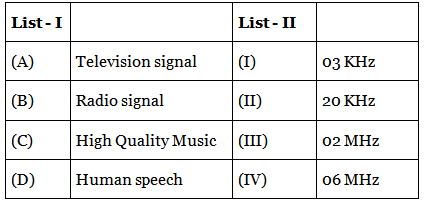(a) A-I, B-II, C-III, D-IV
(b) A-IV, B-III, C-I, D-II
(c) A-IV, B-III, C-II, D-I
(d) A-I, B-II, C-IV, D-III

Ans. c
Television signal ⇒ 6 MHz
High Quality music ⇒ 20 kHz
Human speech ⇒ 3 kHz

Q.21. Amplitude modulated wave is represented by VAM = 10[1 + 0.4cos⁡(2π × 104t)cos⁡(2π × 107t). The total bandwidth of the amplitude modulated wave is : [JEE Main 2022]
(a) 10 kHz
(b) 20 MHz
(c) 20 kHz
(d) 10 MHz

Ans. c
Bandwidth = 2 × fm
= 2 × 104 Hz = 20 kHz

Q.22. The TV transmission tower at a particular station has a height of 125 m. For doubling the coverage of its range, the height of the tower should be increased by [JEE Main 2022]
(a) 125 m
(b) 250 m
(c) 375 m
(d) 500 m

Ans. c
Range R = √2hRe
Let the height be h' to double the range so
2R = √2h′Re
On solving h' = 4h
h' = 500 m
So Δh = 375 m

Q.23. Only 2% of the optical source frequency is the available channel bandwidth for an optical communicating system operating at 1000 nm. If an audio signal requires a bandwidth of 8 kHz, how many channels can be accommodated for transmission : [JEE Main 2022]
(a) 375 × 107
(b) 75 × 107
(c) 375 × 108
(d) 75 × 109

Ans. b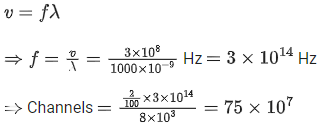Q.24. An antenna is mounted on a 400 m tall building. What will be the wavelength of signal that can be radiated effectively by the transmission tower upto a range of 44 km?
[JEE Main 2022]
(a) 37.8 m
(b) 605 m
(c) 75.6 m
(d) 302 m

Ans. b
h : height of antenna
λ : wavelength of signal
h < λ
λ > h
λ > 400 m

Q.25. A transmitting antenna at top of a tower has a height of 50 m and the height of receiving antenna is 80 m. What is range of communication for Line of Sight (LoS) mode?
[use radius of earth = 6400 km] [JEE Main 2021]
(a) 45.5 km
(b) 80.2 km
(c) 144.1 km
(d) 57.28 km

Ans. d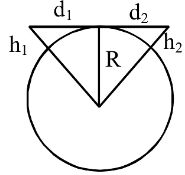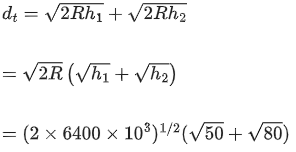= 3578(7.07 + 8.94)
= 57.28 km

Q.26. In amplitude modulation, the message signal [JEE Main 2021]
Vm(t) = 10 sin (2π × 105t) volts and Carrier signal
VC(t) = 20 sin(2π × 107 t) volts
The modulated signal now contains the message signal with lower side band and upper side band frequency, therefore the bandwidth of modulated signal is α kHz. The value of α is :
(a) 200 kHz
(b) 50 kHz
(c) 100 kHz
(d) 0

Ans. a
Bandwidth = 2 × fm
= 2 × 105 HZ = 200 KHZ

Q.27. What should be the height of transmitting antenna and the population covered if the television telecast is to cover a radius of 150 km? The average population density around the tower is 2000/km2 and the value of Re = 6.5 × 106 m. [JEE Main 2021]
(a) Height = 1241 m, Population covered = 7 × 105
(b) Height = 1731 m, Population covered = 1413 × 105
(c) Height = 1800 m, Population covered = 1413 × 108
(d) Height = 1600 m, Population covered = 2 × 105

Ans. b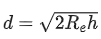Area covered = πd2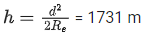Population covered = 2000 × π(d2)

Q.28. A carrier signal C(t) = 25 sin(2.512 × 1010t) is amplitude modulated by a message signal m(t) = 5 sin(1.57 × 108t) and transmitted through an antenna. What will be the bandwidth of the modulated signal? [JEE Main 2021]
(a) 50 MHz
(b) 8 GHz
(c) 1987.5 MHz
(d) 2.01 GHz

Ans. a
β = 2fm(t)
⇒ β = 2 × 1.57×108 / 2π
⇒ β = 50 MHz

Q.29. A 25 m long antenna is mounted on an antenna tower. The height of the antenna tower is 75 m. The wavelength (in meter) of the signal transmitted by this antenna would be :
[JEE Main 2021]
(a) 200
(b) 400
(c) 100
(d) 300

Ans. c
Given that, height of peak of antenna : H = 25 m.
As, we know that
λ = 4H
∴ λ = 4 × 25
⇒ λ = 100 m

Q.30. If a message signal of frequency 'fm' is amplitude modulated with a carrier signal of frequency 'fc' and radiated through an antenna, the wavelength of the corresponding signal in air is : [JEE Main 2021]
(a)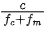(b)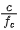(c)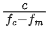(d)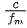Ans. b
Given frequency of massage signal = fm
Frequency of carrier signal = fc
The wavelength of the corresponding signal in air is
⇒ λ =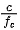Q.31. Given below are two statements : [JEE Main 2021]
Statement I : A speech signal of 2 kHz is used to modulate a carrier signal of 1 MHz. The bandwidth requirement for the signal is 4 kHz.
Statement II : The side band frequencies are 1002 kHz and 998 kHz.
In the light of the above statements, choose the correct answer from the options given below:
(a) Statement I is false but Statement II is true
(b) Both Statement I and Statement II are false
(c) Statement I is true but Statement II is false
(d) Both Statement I and Statement II are true

Ans. d
Side band = (fc − fm) to (fc + fm)
= (1000 − 2) KHz to (1000 + 2) Khz
= 998 KHz to 1002 KHz
Band width = 2fm
= 2 × 2 KHz
= 4 KHz
Both statements are true.

The document JEE Previous Year Questions (2021-22): Communication Systems | Physics 35 Year Past year Papers JEE Main & Advanced is a part of the JEE Course Physics 35 Year Past year Papers JEE Main & Advanced.
All you need of JEE at this link: JEE

## Physics 35 Year Past year Papers JEE Main & Advanced

110 docs|49 tests

## Physics 35 Year Past year Papers JEE Main & Advanced

110 docs|49 tests
Signup to see your scores go up within 7 days! Learn & Practice with 1000+ FREE Notes, Videos & Tests.
10M+ students study on EduRev
Track your progress, build streaks, highlight & save important lessons and more!(Scan QR code)
Related Searches

,

,

,

,

,

,

,

,

,

,

,

,

,

,

,

,

,

,

,

,

,

;